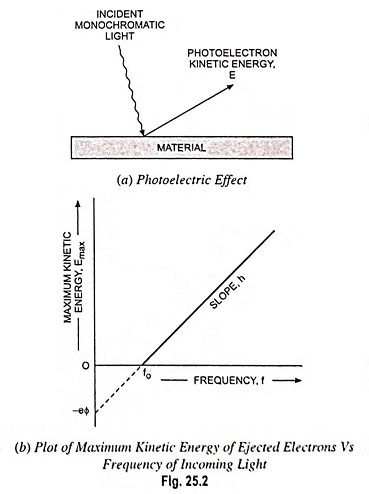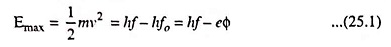## What is Photoelectric Effect?:

When monochromatic light is incident on a clean surface of a material, then under certain conditions, electrons, also called photoelectrons, are emitted from the surface. The electrons in the metal absorb energy from the light, and some of the electrons receive sufficient energy to be ejected from the metal surface into the vacuum. This phenomenon is called the photoelectric effect.

This was Einstein’s experimental interpretation, which involved absorption of optical energy by the electrons in a metal—a relationship depending on energy absorbed and frequency of the light (Fig. 25.2).Optical absorption is the absorption of optical energy as explained above. At a constant incident light intensity, the maximum kinetic energy of the photoelectrons varies linearly with frequency with a limiting frequency f = fo, below which no photoelectron is produced. Fig. 25.2 shows the reflection of photoelectrons and the variations of the maximum kinetic energy with respect to the frequency.

Planck’s experiments in 1900 showed that thermal radiation is emitted from a heated surface in discrete packets of energy called quanta. The energy of these quanta is given by E = hf, where f is the frequency of the radiation and h is a constant now known as Planck’s constant (h = 6.626 x10-34 J-s). A photon with sufficient energy can knock an electron from the surface of the material. The minimum energy required to remove an electron is called the work function of the material and any excess photon energy goes into the kinetic energy of the photoelectron.

The maximum kinetic energy of the photoelectron can be written aswhere v is the maximum velocity of emission of the emitted electrons, hf is the incident photon energy and hfo is the minimum energy, or work function, required to remove an electron from the surface. The quantity Φ (volts) is a characteristic of the particular metal used. When Φ is multiplied by the electronic charge e, an energy in joules is obtained which represents the minimum energy required for an electron to escape from the metal into a vacuum.

Scroll to Top